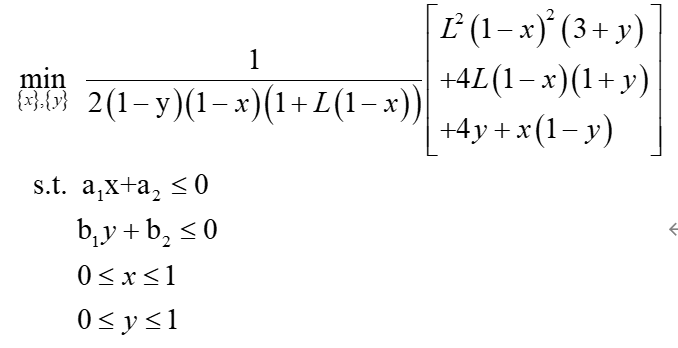# How to solve : Cannot perform the operation: {convex} .* {real affine}I want to use CVX to solve the problem in the first picture. Where x and y are variables and the others are constants. But CVX got it wrong. Please help me find the problem, thank you very much!

The code as follows:

clc
clear all;
L=1;
a1=0.5;
a2=1;
b1=0.5;
b2=0.5;
cvx_begin
cvx_quiet(true)
cvx_solver mosek
variables x y ;

% minimize a1*(-x^2+3*x+2*y^2+3*y+3.5)/(x+1)-a2*y/(x^2-2*x+y^2-8*y+20);
minimize (pow_pos(L,2)*pow_pos(1-x,2)*(3+y)+4*L*(1-x)*(1+y)+4*y+x*(1-y))*inv_pos(2*(1-y)*(1-x)*(1+L*(1-x)));
subject to
x*a1+a2<=0;
y*b1+b2<=0;
x>=0;
x<=1;
y>=0;
y<=1;
cvx_end


The objective function doesn’t look convex. I will assume it is not unless you prove otherwise.

Thank you for your reply. I derive x, y to get the following formula. if0<x,y<1, the formula is >0, so the objective function is convex.

How does that show it’s convex, even over 0 <=x,y <= 1?

When L>0, 0<x<1, 0<y<1, the result of the second partial derivative of the objective function for x, y is greater than zero, and the absolute value of the Hessian matrix is also greater than zero. So the objective function is convex.

You have yet to convince me. Even if your claim is true, if the function is only convex over 0 <= x,y <= 1 rather than over its entire natural domain of -\infty \le x,y \le \infty, it is unlikely there would be a DCP compliant formulation of it.

Now it is convex when 0<x<1, 0<y<1, can I write 0<x<1, 0<y<1 into the constraint and use CVX to solve it?

If you have a DCP formulation, yes? if not, no.

As I wrote above, Even if it is convex, I think it is unlikely there would be a DCP compliant formulation of it.

You can try using YALMIP, and if after reading the tutoial there you are still in need of assistance, you can ask for help on the YALMIP google group.